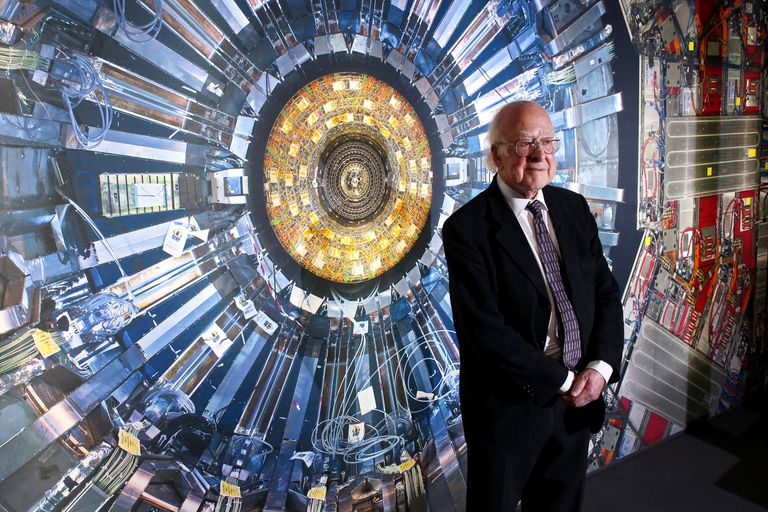### HIGGS MECHANISM AND ITS MISCONCEPTIONSIn my last blog post, ‘working of the large hadron collider‘, I talked about the Higgs boson (famously known as the ‘God particle’) at a certain instant. Today I will be explaining the Higgs mechanism and will unveil some common misconceptions with Higgs boson. I would also introduce some quantum mechanical equations for better understanding.

By 1960s physicist had built a strong understanding of strong nuclear force and the electromagnetic force. Attempts were being made to study weak nuclear force and to combine electromagnetism with weak nuclear force. This attempt is said to make a gauge invariant theory with strong and electroweak force. However, the mathematics predicted two particles the photons (elementary particle of the electromagnetic force) and W, Z bosons (elementary particle of weak nuclear force) to be massless. We did know the photon is massless, however, experiments showed W and Z bosons are not massless bosons. That means the gauge invariant theory is either wrong or incomplete. Other equations which explained the behavior of fermions (electrons, quarks, and neutrinos) with the fundamental non-gravitational forces like strong and weak nuclear force and the electromagnetic force were very consistent and accurate, like the one below: (NOTE: Ψ¯ represents psi with a bar)

(- 1/4)FμνFμν – Ψ¯Ɖ Ѱ

But when we tried to give mass by adding νΨ¯ Ѱ to these matter particles (Ψ¯ Ɖ Ѱ) or even to force-carrying particles ((- 1/4)FμνFμν), the consistency or the symmetry of the equation was shattered away. Soon the physicist Peter Higgs and others introduced a symmetric term ɸ Ψ¯ Ѱ which didn’t look like a mass term at first sight but when you add this term into the equation, you can see the beautiful symmetry of the equation to be maintained.

(- 1/4)FμνFμν – Ψ¯ Ɖ Ѱ + ɸ Ψ¯ Ѱ

The ɸ term can be re-written as ɸ = ν + H and if we multiply Ψ¯ Ѱ on both sides we get:

ɸ Ψ¯ Ѱ = (ν + H) Ψ¯ Ѱ

ɸ Ψ¯ Ѱ = ν Ψ¯ Ѱ + H Ψ¯ Ѱ

And ν Ψ¯ Ѱ is the mass term and ν is the constant vacuum expectation value of the field that is permeating space and H is the scalar Higgs field. So this permeating field in this mathematical term gives rise to the mass of the particles.

This permeating field is the Higgs field which pervades the entire space. After the Big Bang, as the temperature started deducing the unified forces begin to separate. The electroweak force separated as electromagnetic force and weak nuclear force. Therefore, at a certain threshold temperature, the mathematical symmetry of electroweak force starts breaking down as it no longer exists as a single entity but as two separate forces. This is thus called ‘symmetry breaking’ and this breaking of the symmetry is produced by the Higgs Field. The mechanism which led to this symmetry breaking is called the Higgs mechanism.

Higgs field is a scalar (spin-0) field and has a non-zero constant value in the vacuum (non-zero vacuum expectations), similar to an electromagnetic field. The Higgs field is pivotal in generating the masses of quarks and charged leptons (through Yukawa coupling) and the W and Z gauge bosons (through the Higgs mechanism). It is worth noting that the Higgs field does not “create” mass or responsible for the mass of all particles.

Approximately 99% of the mass of baryons (proton and neutron), is due to quark-gluon binding energy (quantum chromodynamics binding energy), which is the sum of the kinetic energies of quarks and the energies of the massless gluons mediating the strong interaction inside the baryons.

In Higgs-based theories, the property of “mass” is a manifestation of potential energy transferred to fundamental particles when they interact (“couple”) with the Higgs field, which had contained that mass in the form of energy. One of the common misconceptions with this field is provided with an analogy where resistance is shown to an object moving in a crowd. But in fact, the Higgs field does not work by resisting motion, it works on the coupling (interaction) with other quantum fields.

There has been considerable scientific research on possible links between the Higgs field and the inflation – a hypothetical field suggested as the explanation for the exponential expansion of space within 10-35 of a second after the Big Bang (inflationary epoch). Some theories suggest that a fundamental scalar field might be responsible for this phenomenon; the Higgs field is such a field, and its existence has led to papers analyzing whether it could also be the inflation responsible for this exponential expansion of the universe during the Big Bang.  So now you know the right description of Higgs boson, do share it with your friends and colleagues and subscribe by clicking the bell button.

1.Yezzbh says: